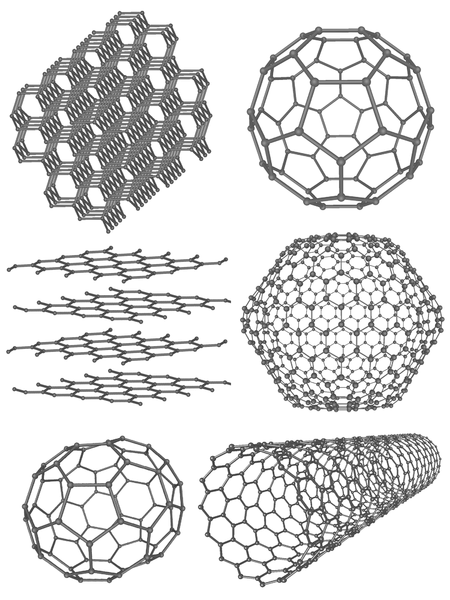# Difference Between Isomorphism and Polymorphism

## Main Difference – Isomorphism vs Polymorphism

In nature, elements and compounds can exist in different combinations, having different structures or morphologies. The structure of a compound determines most of the physical properties and sometimes chemical properties of that compound. The term “morphism” refers to the morphology. It is the external appearance. Therefore, isomorphism and polymorphism are two terms used to describe the morphology of chemical substances. The existence of a substance in more than one crystalline form is known as polymorphism. If this substance is a single element, then it is called allotropy rather than polymorphism. If two or more different substances show the same morphology, then it is called isomorphism. The main difference between isomorphism and polymorphism is that isomorphism describes the presence of the same morphology in different substances whereas polymorphism describes the presence of different morphologies of the same substance.

### Key Areas Covered

1. What is Isomorphism
– Definition, Explanation with Examples
2. What is Polymorphism
– Definition, Explanation with Examples
3. What is the Difference Between Isomorphism and Polymorphism
– Comparison of Key Differences

Key Terms: Allotropy, Isomorphism, Isomorphous, Minerals, Morphology, Polymorphic, Polymorphism## What is Isomorphism

Isomorphism is the similarity in the crystal structure of different compounds. These compounds are called isomorphous substances. In the simplest definition, isomorphous substances are nearly same in their shape.

Isomorphous substances are comprised of the same atomic ratio. Therefore, the empirical formulas of these isomorphous substances are the same. However, since these substances are composed of combinations of different atoms, the chemical and physical properties of isomorphous substances are different from each other. Such properties include mass, density, chemical reactivity, etc.

### Examples of Isomorphism

Some of the examples for substances that show isomorphism are shown below.

• CaCO3 and NaNO3

Both these substances exist in trigonal shape. The atomic ratio of these isomorphous substances is the same (1: 1: 3).

But the physical and chemical properties are different. For example, the molar mass of CaCO3 is 100 g/mol whereas the molar mass of NaNO3 is about 85 g/mol.

• Na3PO4 and N3AsO4

Both substances are tetrahedral in shape. The atomic ratio of both structures is 3: 1: 4. But the physical and chemical properties are different.Figure 01: Different isomorphous compounds are crystallized together to form Pyroxene.

Isomorphism can be often found in minerals. Since the crystal structures are similar to each other, these isomorphous substances can sometimes be crystallized together. Most minerals are composed of this type of structure which has several different compounds mixed together, forming the equal crystal structure. Some of the examples include Feldspar, Garnet, and pyroxene.

## What is Polymorphism

Polymorphism is the presence of different crystalline forms of the same compound. In other words, if a particular compound can be found in different shapes, this phenomenon is called polymorphism. The compound showing polymorphism is called a polymorphic substance.

Polymorphic substances show similarities as well as differences. Most of the times, chemical properties of these polymorphic form of a particular substance is the same, but physical properties can be different. However, the chemical formulas of polymorphic forms are the same since it is the same compound. For example, the CaCO3 compound may exist either in orthorhombic form or in hexagonal form.

When an element show polymorphism, it is called allotropy. When an element is found in different forms of arrangements, those compounds are called allotropes. For example, carbon can be found as either diamond or graphite. They have different chemical and physical properties but are composed only of carbon atoms.Figure 2: Allotropes of Carbon

The above image shows the polymorphism of carbon elements. These different forms or compounds are called allotropes.

## Difference Between Isomorphism and Polymorphism

### Definition

Isomorphism: Isomorphism is the similarity in the crystal structure of different compounds.

Polymorphism: Polymorphism is the presence of different crystalline forms of the same compound.

### Shape

Isomorphism: The crystal shape of isomorphic compounds are identical to each other.

Polymorphism: The crystal shape of polymorphic substances are different from each other.

### Compound

Isomorphism: Isomorphism concerns two or more compounds at a time.

Polymorphism: Polymorphism concerns one compound.

### Elements

Isomorphism: Isomorphism cannot be seen in elements.

Polymorphism: Polymorphism can be observed in elements.

### Atomic Ratio

Isomorphism: The atomic ratios of isomorphous compounds are the same.

Polymorphism: The atomic ratio of polymorphic compounds may or may not be the same.

### Conclusion

Some compounds can be categorized as either isomorphic or polymorphic according to the shape and the composition of the compound. If a particular compound can be found in different shapes, then it is called polymorphism. If different compounds can be found in an identical shape, then it is called isomorphism. The main difference between isomorphism and polymorphism is that isomorphism describes the existence of a same morphology in different substances whereas polymorphism describes the existence of different morphologies of the same substance.

##### References:

1. “Isomorphism.” The Columbia Encyclopedia, 6th ed.. . Encyclopedia.com. Web. Available here. 8 Aug. 2017
2. “Polymorphism.” Encyclopædia Britannica. Encyclopædia Britannica, inc., n.d. Web. Available here. 09 Aug. 2017.

##### Image Courtesy:

1. “SiO3 chains Pyrox vs Wollast” By Solid State – own drawing, created with Diamond 3.1 (CC BY-SA 3.0) via Commons wikimedia (Cropped)
2. “Allotropes of Carbon” By Mstroeck (talk) (Uploads) – Own work (CC BY-SA 3.0) via Commons Wikimedia